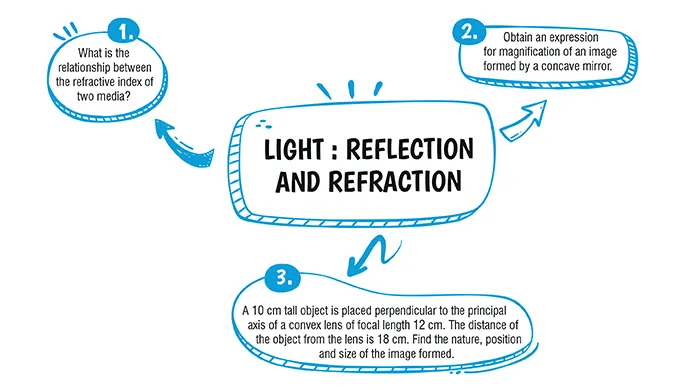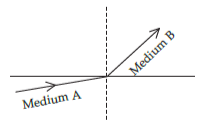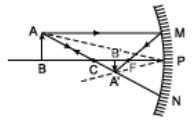Light : Reflection and Refraction

# Class 10 Science Chapter 9 Light : Reflection and Refraction Important QuestionsHere are some critical Chapter 9 Light : Reflection and RefractionProblems for Class 10 Science. These inquiries are intended to aid students in studying for and performing well on the CBSE Class 10 Science Examination 2023–24. Students can clear up their concerns and be ready for the exams by practising different types of questions. By answering these questions, you'll increase your confidence while also sharpening your problem-solving abilities

## Introduction

Chapter 9, "Light: Reflection and Refraction," in Class 10 Science, delves into the captivating phenomenon of light. It encompasses the reflection of light, exploring how it interacts with surfaces, and delves into the intriguing world of spherical mirrors. Additionally, this chapter explores the concept of light refraction, shedding light on how light changes its path when it traverses from one medium to another, unraveling the mysteries of optics and vision.### Class 10 Light : Reflection and Refraction Important Questions and Answers

#### (a) A convex lens of focal length 50 cm.(b) A concave lens of focal length 50 cm.(c) A convex lens of focal length 5 cm.(d) A concave lens of focal length 5 cm.

Ans. (c)

Explanation:
A convex lens with a short focal length (5 cm) is used to read small letters and to see the magnified image of a small object.

#### Q2. A light ray enters from medium A to medium B as shown in figure. The refractive index of medium B relative to A will be:#### (a) greater than unity(b) less than unity(c) equal to unit(d) zero

Ans. (a)

Explanation:
As the light ray when travelled from medium A to medium B, then they bend towards the normal which means that medium B has higher refractive index and less speed of light with respect to medium A, So, refractive index of medium B w.r.t. medium A will be greater than unity.

#### Q3. What is the relationship between the refractive index of two media?

Explanation:
The refractive index for the light going from medium ‘1’ to medium ‘2’ is equal to the reciprocal of the refractive index for light going from medium ‘2’ to medium ‘1’.
1n2 = $$\frac{1}{2^n1}$$

#### Q4. Obtain an expression for magnification of an image formed by a concave mirror.

Explanation:
Consider the formation of the image A′B′ of an object AB by a concave mirror. As shown in the given figure, the right angled triangles ABP and A′B′P are similar triangles, hence
$$\frac{A'B'}{AB} = \frac{A'B'}{AB}$$As per sign convention followed, PB = - u, PB’ = - v, AB = size of the object = + h and A'B' = size of the image = - h’. Hence, we have
$$\frac{-h'}{h} = \frac{-v}{u} or \frac{-h'}{h} = - \frac{v}{u}$$
Thus, by definition of magnification of image, we have
Magnification, m =$$\frac{h'}{h} = \frac{v}{u}$$.

#### Q5. A 10 cm tall object is placed perpendicular to the principal axis of a convex lens of focal length 12 cm. The distance of the object from the lens is 18 cm. Find the nature, position and size of the image formed.

Explanation:
Height of object, h1 = + 10 cm
Focal length, f = + 12 cm
Object distance, u = - 18 cm
From the lens formula,
$$\frac{1}{v} - \frac{1}{u} = \frac{1}{f}$$
⇒ $$\frac{1}{v} - \frac{1}{-18} = \frac{1}{12}$$
⇒ $$\frac{1}{v} + \frac{1}{18} = \frac{1}{12}$$
⇒ $$\frac{1}{v} = \frac{1}{12} - \frac{1}{18}$$
⇒ $$\frac{1}{v} = \frac{3-2}{36} = \frac{1}{36}$$
⇒       v = 36 cm
⇒ Magnification,  m = $$\frac{v}{u} = \frac{36}{-18} = -2$$
The position of the image formed is at a distance of 36 cm from the convex lens.#### CBSE Class 10 Science Chapter wise Important Questions

Chapter No. Chapter Name
Chapter 1 Chemical Reactions and Equations
Chapter 2 Acid, Bases and Salts
Chapter 3 Metals and Non-Metals
Chapter 4 Carbon and its Compounds
Chapter 5 Life Processes
Chapter 6 Control and Coordination
Chapter 7 How do Organisms Reproduce
Chapter 8 Heredity
Chapter 9 Light : Reflection and Refraction
Chapter 10 The Human Eye and the Colourful world
Chapter 11 Electricity
Chapter 12 Magnetic Effects of Electric Current
Chapter 13 Our Environment

#### Conclusion

oswal.io offers a thorough set of questions for learning the topic in a better way if you're looking to further practise and improve your grasp of the concepts covered in the chapter.

#### Q1 : Define the principal focus of a concave mirror.

Ans: Light rays that are parallel to the principal axis of a concave mirror converge at a specific point on its principal axis after reflecting from the mirror. This point is called the principal focus of the concave mirror.

#### Q2: The radius of curvature of a spherical mirror is 20 cm. What is its focal length?

Ans:  Radius of curvature (R) = 20 cm
Radius of curvature of the spherical mirror = 2 × Focal length (f)
R = 2f
f= R/2 = 20 / 2 = 10
Therefore, the focal length of the spherical mirror is 10 cm.

#### Q3 : Name the mirror that can give an erect and enlarged image of an object.

Ans: The mirror that can give an erect and enlarged image of an object is a Concave Mirror.

#### Q4 : Why do we prefer a convex mirror as a rear-view mirror in vehicles?

Ans: A convex mirror is preferred as a rear-view mirror in cars and vehicles as it gives a wider field of view, which helps the driver see most of the traffic behind him. Convex mirrors always form an erect, virtual, and diminished image of the objects placed in front of it.

#### Q5 : A ray of light travelling in air enters obliquely into water. Does the light ray bend towards the normal or away from the normal? Why?

Ans: The light ray bends towards the normal. When a light ray enters from an optically rarer medium (which has a low refractive index) to an optically denser medium (which has a high refractive index), its speed slows down and bends towards the normal. As water is optically denser than air, a ray of light entering from air into water will bend towards the normal.

###### Copyright 2022 OSWAL PUBLISHERS Simplifying ExamsPhone:  (+91) 78959 87722
Mail: support@oswalpublishers.in